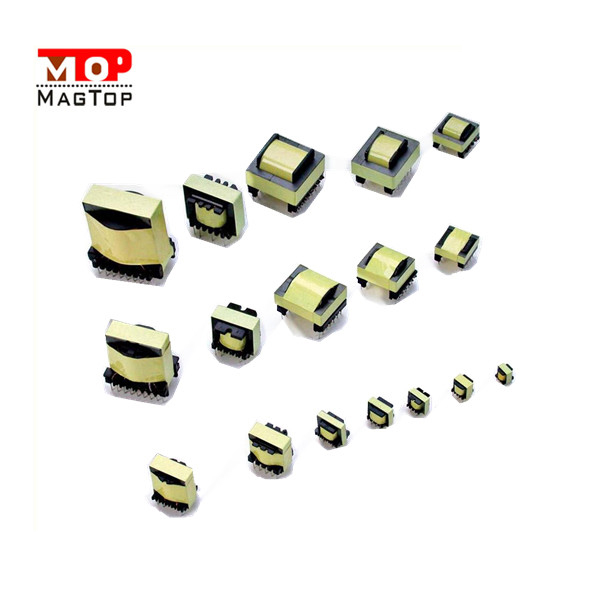# Main parameters of low frequency transformer

##### author: MagTop
25/10/2022Company News | common mode chokes | Industry News | transformer|RJ45 connectors

1. The voltage ratio

Set the number of two groups of low-frequency transformer coils as N1 and N2, N1 is the primary winding, N2 is the secondary winding.
An AC voltage is applied to the primary winding and an induced electromotive force is generated at both ends of the secondary coil.
When N2>N1, its induced electromotive force is higher than the primary voltage added, this low-frequency transformer is called boost low-frequency transformer.

The efficiency of 2.

At rated power, the ratio of the output power to the input power of the low-frequency transformer is called the efficiency of the low-frequency transformer, that is, η=P2/P1×100%.
Where η is the efficiency of the low-frequency transformer, P1 is the input power and P2 is the output power.
When the output power P2 of the low-frequency transformer is equal to the input power P1, the efficiency η is equal to 100%, at this time the low-frequency transformer will not produce any loss, but in fact this low-frequency transformer does not exist, low-frequency transformer transmission power always produce loss, this loss mainly copper loss and iron loss.
The efficiency of the low-frequency transformer is closely related to the power level of the low-frequency transformer. Usually, the greater the power, the smaller the loss and the higher the efficiency.
On the contrary, the lower the power, the lower the efficiency.

3. Working frequency

Because the low frequency transformer core loss and frequency is very important, it should be designed and used according to the frequency of use, this frequency is called the working frequency.

4. Rated voltage

This parameter refers to the voltage allowed to be applied on the primary winding of the low-frequency transformer. The voltage applied on the primary winding of the low-frequency transformer shall not be greater than the specified value in normal operation.

5. Rated power

Rated power refers to the low frequency transformer in the specified frequency and voltage, can work for a long time, and does not exceed the specified temperature rise of the secondary output power.
The unit of rated power of low-frequency transformer is VA(volt ampere), instead of W(watt). This is because there will be part of reactive power in the rated power, so it is more accurate to express VA.

When the low-frequency transformer secondary winding open, there is still a certain current in the primary coil, this current is called no-load current.
The no-load current is composed of magnetizing current (generating magnetic flux) and iron loss current (caused by core loss).
For the low-frequency transformer with 50Hz power supply, the no-load current is basically equal to the magnetization current.

When the low-frequency transformer is open in the secondary, the power loss measured in the primary is the no-load loss.

8. Insulation resistance

This parameter represents the insulation performance between each coil of the low-frequency transformer and between each coil and the iron core.
The insulation resistance is related to the performance, temperature and humidity of the insulating material used.
Insulation resistance is a parameter that represents the insulation performance of low-frequency transformer. It is the ratio of voltage and leakage current applied on the insulation layer, including the insulation resistance value between windings, windings and core and shell.
Because the insulation resistance is very large, generally can only use a megohm meter (or multimeter R×10kΩ block) to measure its resistance.
If the insulation resistance of the low-frequency transformer is too low, the case may be charged in use and even the low-frequency transformer winding may be broken down and burned.

9. The temperature rise of
When the low-frequency transformer is put into operation, the part where the coil temperature is higher than the ambient temperature is called the coil temperature rise.
The part of the core where the operating temperature exceeds the ambient temperature is called the core temperature rise.
There are two kinds of temperature rise: the hottest temperature rise and the average temperature rise.
Generally, the average temperature rise of the coil is taken as the temperature rise index of the low-frequency transformer.
Temperature rise is an important factor affecting the life of low-frequency transformer.
The allowable temperature rise of a low-frequency transformer is determined by its insulation and heat resistance class.